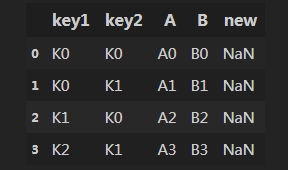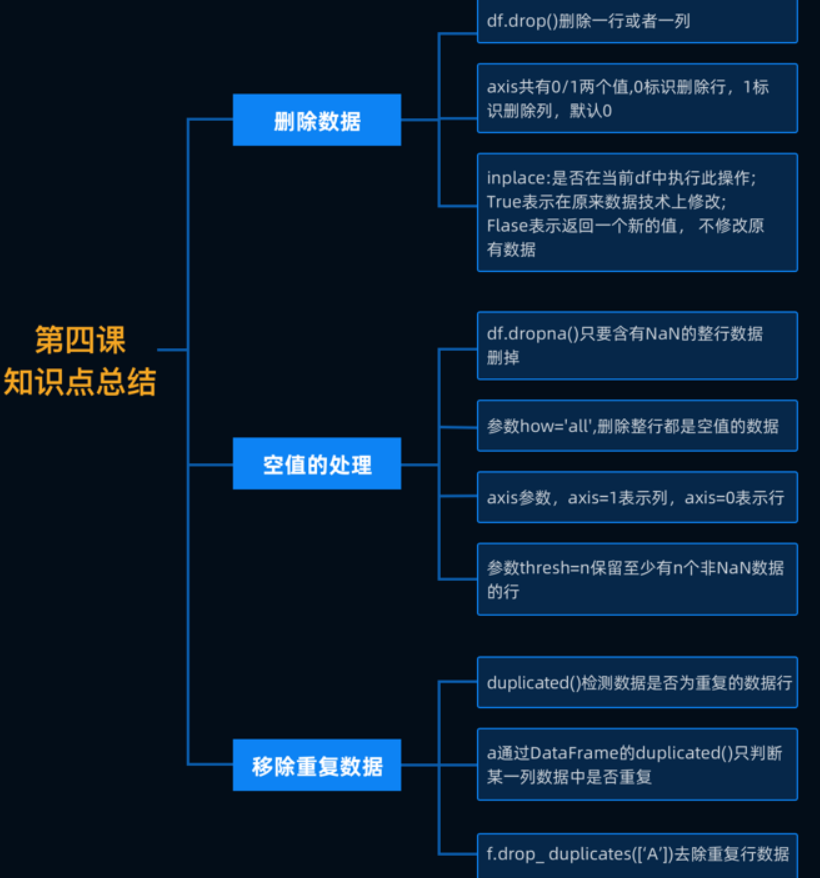• 利用数据框df的name中的非空值，去填充df的features_1中对应的NaN。 很容易写出df[df['features_1'].isnull()]['features_1'']=df[df['features_1'].isnull()][‘name’] ,但是会给出以下警告： A value is ...
利用数据框df的name列中的非空值，去填充df的features_1列中对应的NaN。
很容易写出df[df['features_1'].isnull()]['features_1'']=df[df['features_1'].isnull()][‘name’] ,但是会给出以下警告：
A value is trying to be set on a copy of a slice from a DataFrame. Try using .loc[row_indexer,col_indexer] = value instead
See the caveats in the documentation: http://pandas.pydata.org/pandas-docs/stable/indexing.html#indexing-view-versus-copy
最后发现并没有赋值成功，最后改进为：df.loc[df['features_1'].isnull(),'features_1']=df[df['features_1'].isnull()][‘name’]  ,赋值成功。
参考的链接：https://stackoverflow.com/questions/20625582/how-to-deal-with-settingwithcopywarning-in-pandas

展开全文panda NaN
• 使用pandas，代码如下 const_cols = [c for c in train_df.columns if train_df[c].nunique(dropna=False)==1 ]应该检查标准差，把标准差小的去掉。
使用pandas，代码如下
const_cols = [c for c in train_df.columns if train_df[c].nunique(dropna=False)==1 ]应该检查标准差，把标准差小的去掉。
展开全文• 如何将DataFrame的某一列都变为空值，或者新增一个值为空的列？ 这里需要用到numpy结构，numpy.nan，示例代码如下： import pandas as pd import numpy as np df_test = pd.DataFrame({'key1': ['K0', 'K0', 'K1...
如何将DataFrame的某一列都变为空值，或者新增一个值为空的列？
这里需要用到numpy结构，numpy.nan，示例代码如下：
import pandas as pd
import numpy as np

df_test = pd.DataFrame({'key1': ['K0', 'K0', 'K1', 'K2'],
'key2': ['K0', 'K1', 'K0', 'K1'],
'A': ['A0', 'A1', 'A2', 'A3'],
'B': ['B0', 'B1', 'B2', 'B3']})
df_test['new'] = np.nan
df_test
输出如下图：当列‘new’列存在的时候，会将该列所有值变为NaN；‘new’列不存在的时候，将新建1列，并将该列的所有值变为空值。
展开全文• 1、对于简单的数据删除空值数据（NaN）——对于简单的数据进行过滤，如果对于负责的 DataFrame对象进行使用则会存在空值数据过滤不干净 import pandas as pd——导入模块 li=[2,NaN,4,6,NaN,4]——数据集 se=pd...
                                     第四节  数据七十二变

1、对于简单的数据删除空值数据（NaN）——对于简单的数据进行过滤，如果对于负责的     DataFrame对象进行使用则会存在空值数据过滤不干净
import pandas as pd——导入模块     li=[2,NaN,4,6,NaN,4]——数据集     se=pd.Series(data=li)——生成Series对象     se.notnull()——对是否是空值数据进行判断（返回值是bool值）     print(se[se.notnull()])——返回过滤之后的数据
2、删除空值数据（NaN）的行和列
使用dropna函数：         df1=df.dropna()
dropna()是删除空值数据的方法，默认将只要含有NaN的整行数据删掉，如果想要删除整行都是空值的数据     需要添加how='all'参数。     如果想要对列做删除操作，需要添加axis参数，axis=1表示列，axis=0表示行。     我们也可以使用thresh参数筛选想要删除的数据，thresh=n保留至少有n个非NaN数据的行。
3、删除数据
使用函数drop(labels=None,axis=0, index=None, columns=None, inplace=False)         labels ：就是要删除的行列的名字，用列表给定。         axis：axis=1表示列，axis=0表示行         index： 直接指定要删除的行。         columns： 直接指定要删除的列。         inplace=False：默认该删除操作不改变原数据，而是返回一个执行删除操作后的新dataframe。         inplace=True：则会直接在原数据上进行删除操作，删除后无法返回。     ///         DataFrame.drop(labels=None,axis=0, index=None, columns=None, inplace=False)
总结：最后总结出删除数据可以使用两种方式：         （1）使用labels参数和axis参数结合来删除数据         （2）使用index或者是columns来删除参数，而且index和columns可以同时使用，但是同时使用时             删除的不是某一个单元格，而是所在行和所在列都会删除
4、空值的处理（NaN表示空值）
对于空值我们可以将整条数据删除，也可以使用fillna()方法对空值进行填充
df.fillna(value=None, method=None, axis=None, inplace=False, limit=None, downcast=None, **kwargs)
注意：method参数不能与value参数同时出现。
填充方法有以下三种：         （1）使用常数填充             df.fillna(常数)         （2）使用列的平均数填充             df.fillna(df.mean())         (3)使用前面前面一个值进行填充             df.fillna(method="ffill",axis=0)
5、重复数据的处理     重复数据的存在有时不仅会降低分析的准确度，也会降低分析的效率。所以我们在整理数据的     时候应该将重复的数据删除掉
判断是否是重复数据：         利用duplicated()函数返回的是一个值为Bool类型的Series，如果当前行所有列的数据与前面         的数据是重复的就返回True；反之，则返回False             print(df.duplicated())
删除重复数据         可以使用drop_duplicates()将重复的数据行进行删除             df.drop_duplicates()         也可以对列数据进行判断，然后把重复的数据删除             df.drop_duplicates([列名],inplace=False)
总结展开全文python
• 主要介绍了Python实现删除中含有空值的行的示例代码，文中通过示例代码介绍的非常详细，对大家的学习或者工作具有一定的参考学习价值，需要的朋友们下面随着小编来一起学习学习吧
• 要求：将number_1中的空值用number_2中的非空值进行填充。方法如下： > df [ "number_1" ] . fillna ( df [ "number_2" ] , inplace = True ) > print ( df ) name number_1 number_2 0 apple 1.0 NaN ...
• 删除数据 二.空值的处理 df.fillna(value=None,method=None,axis=0,inplace=False,limit=None,downcast=None,**kwargs) value:填充空值 method:{bfill、backfill、ffill、pad、None} bfill、backfill填充前...
• 假设拿到个10万行的数据后，通过isnull我们发现某有几个空值，要把该列空值所在行删除怎么操作？用dropna()会删除所有有空值的行，请看下面实例。 ...pandas DataFrame
• 表格排序列的数据出现空值时，组件自带的排序功能不正常。而后查找了相关解决方法，有网友给了我启示，解决了问题，现在照着思路写下步骤，日后再次遇到方便查找 解决方式是重新定义个新数组：遍历表格数据...vue.js javascript
• df['数值']=df['数值'].fillna(df.mean()['数值1']) 替换前 替换后python数据库 .net null
• mydf['列名']=mydf['列名'].fillna(0)pandas
• 可以给字段的值设置成0、个特殊的值或者个空串代替空值。 MySQL难以优化引用了可空的查询,它会使索引、索引统计和值更加复杂。 可空需要更多的储存空间，还需要在MySQL内部进行特殊处...
• 在spark dataset/dataframe操作过程中，我们经常会遇到对于值的一些判断情况，是否为NULL，创建个新等，本文讲解了常用的增加的方法，并且对于列空值判断，填充处理以及查询的api做了详细的描述和实例...spark
• Sub Del() num = ActiveSheet.UsedRange.Rows.Count() For i = 1 To num If Cells(i, 9) = "" Then Rows(i).Delete End If Next End Sub
• 把df中a空值用b的值做补充 df['a'][df['a'].isnull()] = dfdf['b'][df['a'].isnull()]
• 1、删除未经处理的空值数据所在的行或 在这种情况下我们看到的空值数据通常显示的是NaN 那么这种情况就比较好处理直接调用dropna()函数 应用： order.dropna(axis=1) 删除过后的样子： 2、删除已用代表空值的...
• ## Python实现删除某列中含有空值的行

万次阅读 多人点赞 2019-08-30 13:53:47
查看销售人员不为空值的行 数据存储情况如图： 代码实现 import pandas as pd data = pd . read_excel ( 'test.xlsx' , sheet_name = 'Sheet1' ) datanota = data [ data [ '销售人员' ] . notna ( ...
• 万水千山总是情，点个关注行不行 加微信 yyf1752432501 领取python免费资料python pandas dataframe
• mydf['列名']=mydf['列名'].fillna(0)
• 有时我们用pandas读取Excel去获取某或者某几列的值，并需要对该的值进行处理，首先要判断该是否有空值，若有空值不做判断可能程序会报错，比如用正则表达式去匹配该的所有单元格的值，这时若某个单元格为...python excel
• 本文整理了数据空值的处理操作...删除空值 dropna()函数 填充空值fillna()函数 为了便于描述，定义本文示例数据为如下结构： df = pd.DataFrame([[1, np.nan], [np.nan, 4], [5,6],[np.nan,7]],columns=["A",...python pandas numpy
• ## pandas 删除某列空值

万次阅读 2018-05-15 17:53:39
import pandas as pd # 删除含有空数据的全部行 ...# 可以通过axis参数来删除含有空数据的全部 df4 = df4.dropna(axis=1) # 可以通过subset参数来删除在age和sex中含有空数据的全部行 df4 = df4.dr...
• 处理丢失数据 有两种丢失的数据： -- None -- np.nan(NaN) 1.None None是Python自带的，其类型为python object。因此，None不能参与到任何计算中 object类型的运算要比int类型的运算慢得多 2.np.nan...DataFrame 处理缺失值
• df.info(verbose=True， null_counts=True)
• 会尽可能把一些重要技术知识点写入开头，增加阅读体验，另外我会把工作中遇到的实际问题，写成文章发布，比如今天这个删除样本空值，还有昨天用python解方程，都是我在数据分析工作中遇到的需求，对于每个技术点，...
• 创建测试dataframe： >>> import pandas as pd >>> df = pd.DataFrame([{'a':1, 'b':...添加两个空 >>> df[['c','d']]=df.apply(lambda x:('',''),axis=1,result_type='expand') >>pandas python dataframe
• A是#N/A，则B不是空值空值替换成正常值，即两列合并成一 =IF(ISNA(A2),B2,A2)
• ## R语言读取数据空值

万次阅读 2016-09-30 10:32:12
、Txt文件 1. 文件中的内容有双引号，空值的时候是一对双引号 filename.txt 文件内容形如： "ID" "ITEM" "1080254842" "汉字" "1080254842" "中文" "1080594798" "" "1080594798" "" 导入数据： test ...na...